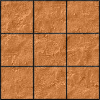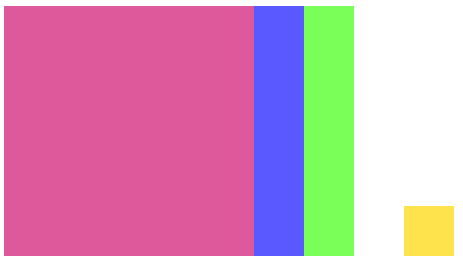#### You may also like### Pyramids

What are the missing numbers in the pyramids?### Paving the Way

A man paved a square courtyard and then decided that it was too small. He took up the tiles, bought 100 more and used them to pave another square courtyard. How many tiles did he use altogether?### Chess

What would be the smallest number of moves needed to move a Knight from a chess set from one corner to the opposite corner of a 99 by 99 square board?

##### Age 11 to 14 Challenge Level:

Charlie has been playing with calculations again...

$2 \times 4 + 1 = 9$
$4 \times 6 + 1 = 25$
$5 \times 7 + 1 = 36$
$9 \times 11 + 1 = 100$

What do you notice?

Click below to see what Charlie said:

"If you multiply two numbers that differ by 2, and then add one, the answer is always the square of the number between them!"

Can you explain what's happening?

Click below to see Charlie's explanation:

$n(n+2) +1 = n^2 +2n+1 = (n+1)^2$
or alternatively,
$(n-1)(n+1)+1 = n^2-n+n-1+1=n^2$

Alison drew a diagram to explain the results. Click below to see:Can you make sense of Charlie's method and Alison's diagrams?

Here are some more number patterns to explore. Some have been expressed numerically, some in words, and some algebraically.

Can you represent each pattern in all four ways,
numerically (so you can spot the pattern),
in words (so you can describe the pattern),
algebraically (so you can prove the pattern continues),
and using a diagram (to explain the pattern)?

1. $2 \times 3 + 3 = \, ?$
$5 \times 6 + 6 = \, ?$
$4 \times 5 + 5 = \, ?$
$9 \times 10 + 10 = \, ?$
What do you notice?

2. Choose three consecutive numbers, square the middle one, and subtract the product of the other two.
Repeat with some other sets of numbers.
What do you notice?

3. $3 \times 3 - 1 \times 1 = \, ?$
$8 \times 8 - 6 \times 6 = \, ?$
$7 \times 7 - 5 \times 5 = \, ?$
$10 \times 10 - 8 \times 8 = \, ?$
What do you notice?

4. $n(n+1) - (n-1)(n+2) = \, ?$
$(n+1)(n+2) - n(n+3) = \, ?$
$(n-3)(n-2) - (n-4)(n-1) = \, ?$
What do you notice?

5. $3\times 5 + 1= \, ?$
$5\times 7 + 1= \, ?$
$7\times 9 + 1= \, ?$
$9\times 11 + 1= \, ?$
What do you notice?

6. Choose three consecutive numbers and add the product of the smallest two to the product of the greatest two.
Repeat with some other sets of numbers.
What do you notice?

With thanks to Don Steward, whose ideas formed the basis of this problem.